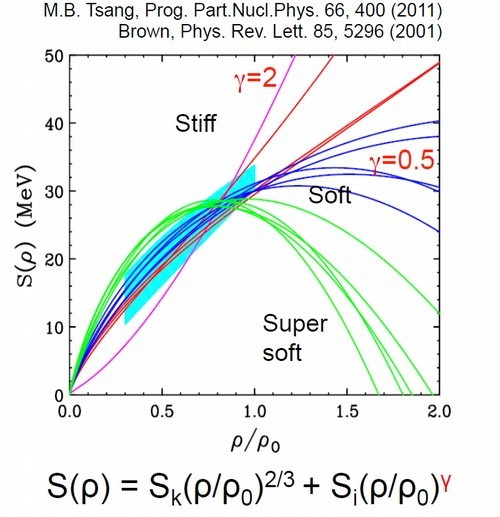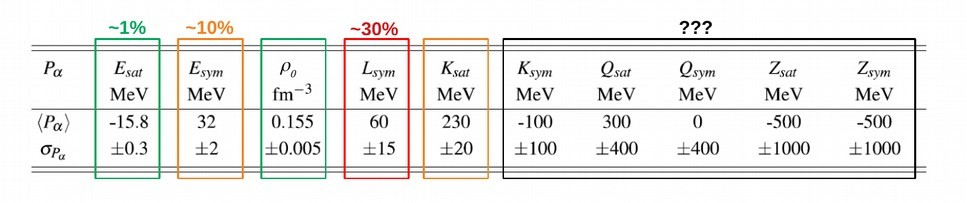# Nuclear physics

## The equation of state in the astrophysical context

The equation of state of nuclear matter is also crucial for the description of compact stellar objects like neutron stars in the astrophysical context. In this case, it is necessary to reach the largest possible ratio of neutrons N to protons Z (isospin N/Z) as found in neutron stars. This is why one of the current challenges of the field is the study of the isovector dependence (isospin) of the equation of state, which is described by the symmetry energy. The symmetry energy is indeed the energy necessary for a nuclear system to go from an equal ratio between neutrons and protons (symmetric matter) to a matter composed only of neutrons (neutron matter). This quantity is currently poorly constrained by experimental data as well as models, in particular with respect to its density dependence. Figure 2 presents different existing theoretical predictions compared to experimental results. It can be seen that the uncertainties become very large for the high density regime (ρ>1) but also at intermediate density (0.2<ρ<0.7).Dépendance en densité de l'énergie de symétrie S(ρ). La densité est ici exprimée en fraction de la densité de saturation ρ0. Les différentes courbes montrent les prédictions théoriques attendues. La zone en turquoise donnent les contraintes obtenues expérimentalement. Extrait de B. Brown, Physical Review Letters 85 (2001).
The current goal of the Nuclear Dynamics and Thermodynamics team is to try to provide the most accurate experimental constraints, allowing to reach an uncertainty on the isovectoral part (in particular on the Lsym term of the symmetry energy) of the equation of state lower than 10% against 30% at present. Table 1 presented below gives the current estimates <Pα> as well as the known uncertainties &#916Pα with respect to the different terms of the equation of state of nuclear matter. Note that 2 estimates, Esat and ρ0 are very well known (1%), while all others suffer from uncertainties ranging from 10% to 200% for some.Tableau 1: Estimations moyennes <Pa> et incertitudes associées sPa des différents termes de l’équation d’état connues actuellement. Extrait de J. Margueron et al., Physical Review C 97, 025085 (2018).

The precise characterization of the equation of state of nuclear matter requires the study of collisions between nuclei (heavy ions) around the Fermi energy (EFermi = 38 MeV per nucleon). In this case, the atomic nuclei are characterized by the equation of state which represents the bulk term, supplemented by a term related to the finite surface of the nuclei and the Coulombic interaction between protons. The study of collisions also provides experimental constraints on the dynamics of the collision (transport and equilibration properties) as well as the thermodynamics of the nuclear systems produced (phase diagram of nuclear matter and equation of state). The beams used come mainly from the accelerator facilities GANIL in Caen (France), GSI in Darmstadt (Germany) and LNS in Catania (Italy), which are able to deliver projectile beams in the incident energy range around the Fermi energy and between 20 and 100 MeV per nucleon. The use of different combinations of projectile and target beams allows to vary the amount of energy, the mass of the ephemeral nuclear systems created during the collisions, as well as the ratio between the number of neutrons and protons (isospin). The experimental device used since 1993 is the INDRA multidetector (collaboration including 4 laboratories of IN2P3), through data collected for more than 25 years from GANIL (stable beams and SPIRAL) as well as from GSI in Germany. The experimental program continues since 2019 thanks to the contribution of a new multidetector called FAZIA in the framework of an international collaboration.

Scroll to top